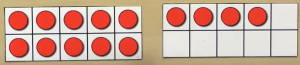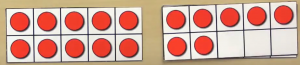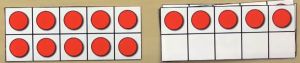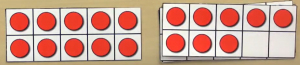# Lesson 4: Addition up to 20 with worksheets

Lesson four ten and another Now that students are comfortable with ten frames, we introduce the concept of ten and another. Here without counting one by one we can tell that we have four items. If we put a full 10 frame in front of it without counting one by one, we can tell that we have 14 items.Now we have 17 items.Next is 15 items,and finally 18 items.Notice how we did not have to count one by one. Let’s take a look at the assignment. Simply write down the number of items in each row without counting one by one. The example row shows 14 items. Have students practice saying the answers aloud first. For example, here we have 17, 11, 20, and 13 items. When students are comfortable they should write down their answers and they should try to finish this section in less than 45 seconds. Here we have 17, 20, 11, and 13.

## Worksheets for the lessonМатематичні настільні ігри